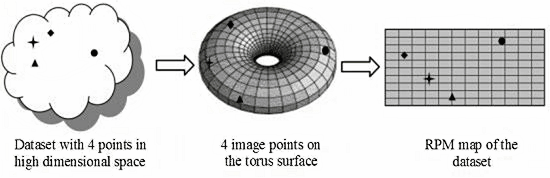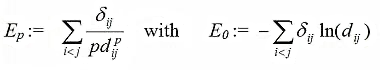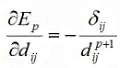See the Invisible in your DataA Brief Introduction to Relational Perspective Map Relational perspective map (RPM), developed by James X. Li,  is a general purpose method for visualizing distance information of data points in high dimensional spaces. The starting point of the RPM algorithm is a set of data point si, i=1,...,N, and a distance matrix δij. The matrix δij, called the relational distance, is the numeric representation of a relationship between the data points. The goal of the RPM algorithm is to map the data points si into a two or three dimensional map so that Euclidean distances dij between the image points visually approaches δij as much as possible. The resulting lower dimensional map is called Relational Perspective Map, the matrix dij is called the Image Distance Matrix. From geometric point of view, an RPM map attempts to preserve as much as possible the distance information within the original dataset. The following figure demonstrates how the RPM algorithm works to create 2D maps: it first maps data points to the surface of a torus, then unfolds the torus surface by a vertical and a horizontal cut. This second step is relatively straightforward (see here for a short video showing the transformation between rectangle and torus), the innovation of the RPM algorithm lies in mapping the data points to the torus surface so that the distances between the image points resembles the distances between data points.In order the find the best mapping, the RPM algorithm defines an energy function as follows:where p is an algorithm parameter called the rigidity, dij  is the geodesic block distance between two image points on the torus surface. The RPM algorithm then uses gradient descent optimization method to find the lowest-energy configuration. The rigidity parameter, which is normally a value between -1 and +1, alters the energy landscape in a global manner, so that the resulting RPM maps have different characteristics. To better understand the RPM algorithm it is helpful to consider the image points on the torus as a force-directed, multi-particale system with mutual repulsive forces between them; and consider the energy Ep as a kind of total potential energy. According to physics, the repulsive force is characterized as proportional to relational distance:The optimization strategy is to minimize the energy Ep by simulating a dynamic system directed by the force given by above form. Since points with larger relational distances between them correspond to larger repulsive force on the torus, their image points on the torus should  be further apart from each other. The key idea of RPM algorithm is its exploitation of the properties of closed manifold (the torus) to keep the configuration in balance. (Competing approaches are identified in the next section.) Whereas other non-linear methods apply, directly or indirectly, attractive force to map closely related points to closely located positions,  the RPM algorithm maps closely related points within a closely located area by modeling the collective repulsive force of all points. This approach makes RPM a true, and (as far as we know) the only, global mapping algorithm. It should be noted here that the RPM algorithm relaxes one condition of the original problem setting: the resulting map is not a normal rectangle, but a torus. This means that the opposite edges of the map should be considered as contiguous or joined. Related Methods: Visualizing high dimensional data has been a major topic since many decades. A large group of methods target high dimensional data with sophisticated rendering techniques like 3D landscapes, special glyphs, colors, and graphics etc. Other methods approach the problem by reducing the dimensionality in a generic way with few assumptions about data type. RPM algorithm belongs to the latter group. The following is a short list of methods that directly or indirectly reduce data dimensionality: Multidiemensional Scaling: Sammon Mapping, Curvilinear Component Analysis. Self-Organzing Map. Principal Component Analysis/Singulare Value Decomposition. Non-linear Projections:  Local Linear Embedding. Methods based on physical models: spring embedding model; force directed placement. References: James Xinzhi Li: Visualization of High Dimensional Data with Relational Perspective Map. Information Visualization 2004, Vol. 3, No. 1. 49-59.